# First Flight Simulator Program: FlightSimulator

## First Flight Simulator Program: FlightSimulator

We will begin with a simple program that imports an F16 aircraft from the Jamagic CD-ROM and rotates the aircraft along the x and z axes, while displaying the amount of rotation on the toolbar. We will also display the sine and cosine of the angle of roll. The sine and cosine are super-important trigonometric functions that you really should understand fully. We will use them in the next project to make the aircraft move to one side faster the more it rolls, and to calculate the vertical speed of the aircraft when it s moving pitched up or down.

If you have never had any trigonometry and are serious about learning to program games , the following explanations of sine and cosine will be worth your time.

### Sine and Cosine

Let s say that we have a circle in 2D space with it s center at (0,0), as shown in Figure 5.3. So we can refer to it later, we will call this circle the unit circle . The radius of the circle (the distance from the center point to the edge of the circle) is 1. You can see on the figure that the circle crosses the x-axis at x = 1 and x = “1, and it crosses the y-axis at y = 1 and y = “1. Notice that the negative y-axis is up, while the positive y-axis is down! This is different from what you may be used to, and it goes along with the convention used for screen coordinates. Recall that the screen origin is at (0,0) and y values become greater as you go down the screen.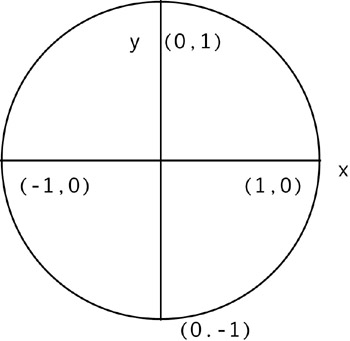Figure 5.3: The unit circle.

Let s draw an angle of 30 degrees on our unit circle, measured from the x-axis (see Figure 5.4). Remember that 2 pi (pi = 3.1417) radians correspond to 360 degrees, so to convert from degrees to radians multiply the degrees by 0.01745 ”30 degrees is then 0.524 radians.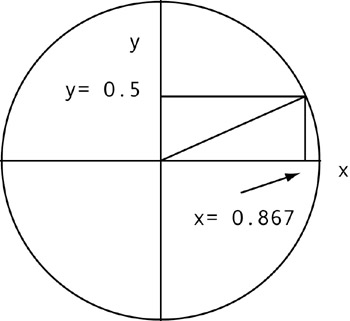Figure 5.4: 0.524 radians (30 degrees) on the unit circle. Cosine is 0.867 and sine is 0.5.

In Figure 5.4, you will notice that we have drawn a vertical line to the x-axis and a horizontal line to the y-axis from the point where the angle meets the circle. You can see that the vertical line hits the x-axis at 0.867, and the horizontal line hits the y-axis at 0.5. The value where the vertical line hits the x-axis is called the cosine, and the value where the horizontal line hits the y-axis is called the sine. That s all there is to it.

Imagine what happens to the sine and cosine as we increase the angle to 45 degrees: the vertical line shifts to the left and the horizontal line moves down. At 45 degrees, the sine and cosine equal each other at a value of 0.707. So, as we go from 0 degrees to 90 degrees, it looks as if sine is increasing and cosine is decreasing .

Beyond 90 degrees the patterns changes and the cosine begins increasing again (although it has a negative value after 90 degrees) as the sine decreases.

Let s try to come up with some sines and cosines for some easy angles. Pleases consult the Figure 5.4 so that you can envision what is happening. At an angle of 0 degrees (0 radians) to the x-axis, the cosine is 1 and the sine is 0. At an angle of 90 degrees (pi/2 = 1.57 radians), the cosine is 0 and the sine is 1. At an angle of 180 degrees (pi = 3.14 radians) the cosine is “1 and the sine is 0. At an angle of 270 degrees (3/2 pi = 4.7 radians) the cosine is 0 and the sine is “1. At an angle of 360 degrees (2 pi radians = 6.28 radians) the cosine is again 1 and the sine is 0 (in other words, 0 and 360 are the same!). For angles greater than 6.28 radians we begin counting from zero again.

Your question right now may be, OK, this isn t so difficult, but why bother with sine and cosine? Remember that one of the features of our game will be to have the jet move faster to the side as the roll angle increases , which is a very realistic feature. We need some formula to calculate the sideways speed of the jet based on the angle, and the sine function will be extremely useful for this. Remember, the value of the sine is increasing from a value 0 at 0 degrees to a value of 1 at 90 degrees. If we take a number, say 100, and multiply it by the sine of the angle of roll, we get a pretty good pattern forming for sideways airspeed!

Basically, this is the way it works. At zero roll,

` 100 * sin 0 = 0, `

so sideways speed is 0. At a 45 degree roll,

` 100* sin 45 = 100*.707 = 70.7. `

The sideways speed is then 70.7. At a 90 degree roll,

` 100 * sin 90 = 100, `

so our sideways speed is at a maximum value of 100.

Pretty good, huh? We will use this in the very next program. In this program, you should study what happens on the screen to the sine and cosine as the roll angle changes.

Figures 5.5 through 5.10 show the jet through an entire 360 degree rotation (2 pi) about the z-axis, with the aircraft seen from the front. In order to understand what we mean when we say that the aircraft is rotating about the z-axis, imagine that the jet is mounted on a shaft that runs through its length. Notice what happens to the sine and cosine.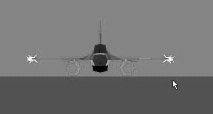Figure 5.5  :  Jet seen from the front in level flight. The z-axis points into the viewer. The x-axis runs horizontally. They y-axis runs vertically. Sine is 0.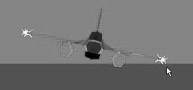Figure 5.6  :  Jet is rotated 0.196 radians about the z-axis. Sine is 0.195.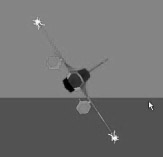Figure 5.7  :  Jet is rotated 0.983 radians about the z-axis. Sine is 0.83.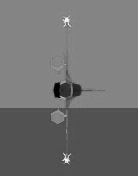Figure 5.8  :  Jet is rotated 1.57 radians about the z-axis. Sine is 1.0.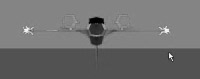Figure 5.9  :  Jet is rotated 3.148 radians about the z-axis. Sine is 0.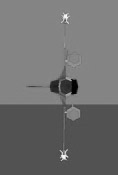Figure 5.10  :  Jet is rotated 4.72 radians about the z-axis. Sine is “1.

We will also pitch the jet up and down, again using radians to measure the angle. The aircraft rotates about its x-axis when it is pitching; imagine that the jet is rotating about a shaft that runs parallel to its wingspan. Figures 5.11 and 5.12 show rotation about the x-axis at pi/4 and pi/2 radians (pointing straight down).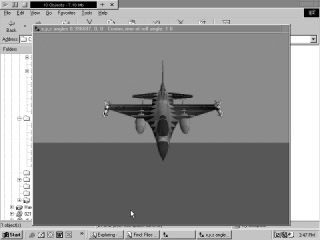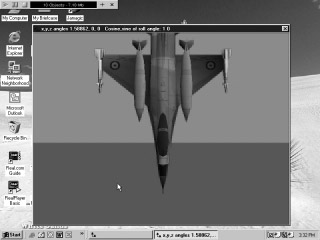Figure 5.12  :  Jet rotated pi/2 radians (straight down) about the x-axis.

You have seen us rotate the jet about the x- and z-axes. Of course, we could also have done the same with the y-axis (yaw), for a total rotation about all three axes. We can describe the total rotation of an object by specifying the rotation about all three axes. For example, an object rotated pi/4 radians about the x-axis, pi/2 radians about the y-axis, and pi radians about the z-axis could be described by the expression (.785, 1.57, 3.14).

### Sine and Cosine Demonstration Program

We first want to get a feel for the sine and cosine functions, so we will make a demonstration program,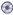FlightSimulator , that rotates the jet about different axes in pitch and roll while displaying the sine and cosine of the roll angle. In fact, the images you saw in the previous section were created using this demonstration program.

We will roll and pitch the aircraft using the arrow keys and the RollLeft , RollRight , TurnDown , and TurnUp commands in Jamagic . When the control stick is pushed forward the plane dives, and when it is pulled back the plane pitches up. For this reason, we would like the aircraft to dive when the up arrow key is pressed, and pitch up when the down arrow key is pressed, which may at first seem counter-intuitive. Even more confusing is that the TurnUp command makes the aircraft dive!

Please see the following code for an example of how we make the aircraft respond to the arrow keys; the amount of rotation in radians is first specified in the parentheses, followed by the number of seconds the motion is to last. In each case, the rotation is set to pi/16 radians (11.25 degrees). This will be changed in the next program.

` If(Keyboard.IsKeyDown(Keyboard.LEFT))  {    myjet.RollLeft(Pi/16,1);  } If(Keyboard.IsKeyDown(Keyboard.RIGHT))  {    myjet.RollRight(Pi/16.,1);   } If(Keyboard.IsKeyDown(Keyboard.UP))  {    myjet.TurnUp(Pi/16,1);   } If(Keyboard.IsKeyDown(Keyboard.DOWN))  {    myjet.TurnDown(Pi/16.,1);   } `

We also wish to display the rotation about each axis, as well as the sine and cosine of the roll angle. We do so with the following command, which sends text to the window s toolbar area:

` // next all on one line! SetText("x,y,z angles "+ myjet.GetXAngle()+ ", "+myjet.GetYAngle() + ", "+myjet.GetZAngle()+ " Cosine,sine of roll angle: " + Cos(myjet.GetZAngle) + " "+Sin(myjet.GetZAngle));// last 4 lines on one // line `

There is only one other new command used in this program; it is used to set a sky-colored background on the screen:

` ocamera1.SetBackgroundColor(GetRGB(0,150,200)); `

When the jet is loaded into your program, it will be loaded in facing you ”the direction it faces when loaded depends on how it was drawn. Since the camera always faces from the negative direction to the positive z direction, we will need to place our camera closer to the viewer to make sure the jet is visible.

You can now type in the code. You should understand the remaining portions from the previous chapters. When you run the program, please note how the x and z angles and sine and cosine change as you rotate the jet.

### Sine and Cosine Demo Code: FlightSimulator

` owindow1 = New Window("Flight Simulator",640,480,Window.STANDARD);//one line //Window maintenance owindow1.SetAutoRefresh(OFF); // New world oworld = New World(); //Import jet. oworld.Load("f16"); myjet= oworld.GetObject(oworld.GetNObjects()1); myjet.Scale(0.01); myjet.SetPosition(0,150,0); // Create the ground surface and rotate it so it's flat. ground = oworld.CreatePlane(10000,10000); ground.SetPosition(0,0,0); ground.SetStatic(); ground.SetAngle(Pi/2,0,0); ground.SetStatic(); matp =New Material(oworld,GetRGB(0,100,50)); matp.SetFlat(ON); ground.ReplaceMaterial(matp); // New cameras ocamera1 = New Camera(oworld,owindow1); ocamera1.SetPosition(0,150,75); ocamera1.SetBackgroundColor(GetRGB(0,150,200)); oworld.Optimize(ocamera1); //Game loop While(1) {     ocamera1.ActivateRender();     owindow1.Refresh(); If(Keyboard.IsKeyDown(Keyboard.LEFT))  {    myjet.RollLeft(Pi/16,1);  } If(Keyboard.IsKeyDown(Keyboard.RIGHT))  {    myjet.RollRight(Pi/16.,1);   } If(Keyboard.IsKeyDown(Keyboard.UP))  {    myjet.TurnUp(Pi/16,1);   } If(Keyboard.IsKeyDown(Keyboard.DOWN))  {    myjet.TurnDown(Pi/16.,1);  }  SetText("x,y,z angles "+ myjet.GetXAngle()+ ", "+myjet.GetYAngle() + ", "+myjet.GetZAngle()+ "   Cosine,sine of roll angle: " + Cos(myjet.GetZAngle) + " "+Sin(myjet.GetZAngle));//all      // on one line //End of game loop follows. } `

Experiment thoroughly with the program. Observe what happens to the sine and cosine values of the roll angle until you get very comfortable with what happens as the roll angle changes. Once you are ready, you may progress to the next program, which will be a working flight simulator.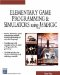Elementary Game Programming & Simulations Using Jamagic (Charles River Media Game Development)
ISBN: 1584502614
EAN: 2147483647
Year: 2002
Pages: 105
Authors: Sergio Perez